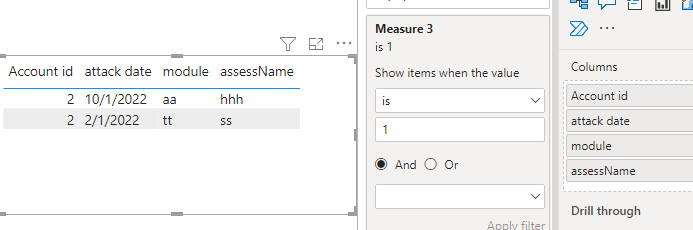cancel
Showing results for
Did you mean:Frequent Visitor

## count with condition

Hi All,

this is my data sample:

 Account id attackid attack date module assessName status 1 01 1/1/22 aa xx failed 1 02 2/1/22 aa yy failed 1 03 2/1/22 cc xx failed 1 04 3/1/22 aa xx completed 2 05 1/1/22 tt ss failed 2 06 2/1/22 tt ss failed 2 07 2/1/22 aa hhh failed 2 08 10/1/22 aa hhh failed 3 09 1/1/22 rr pp failed 3 10 2/1/22 aa mm failed 3 11 8/1/22 rr pp failed

i'm trying to disply a table that will give me all account that runs 2 failed attakcs in row

the attack must to be in a row and must to have same assessName and same module name

for example account 2 had 2 failed attacks in a row with the same module = tt and the same assessName = ss

acount can answer this conditions correctly more then 1 time,

for example account 2 add another 2 failed attacks in a row with the same module = aa and the same assessName = hhh

i want to disply on the report a table with all the attacks that answer these conditions

 id Last attack date module assessName 2 2/1/22 tt ss 2 10/1/22 aa hhh

and then count the number of attacks that  answer these conditions (count shold be 2 base on the sample data)

can it be done only with maesure and not with calculated column/table ?

pls help 🙂

1 ACCEPTED SOLUTIONCommunity Support

Hi, @ReutAtias12

Of course.

Measure:

``````Measure 1 =
CALCULATE (
COUNT ( 'Table'[Account id] ),
FILTER (ALL('Table'),
[assessName] = SELECTEDVALUE( 'Table'[assessName] )
&& [module] = SELECTEDVALUE( 'Table'[module] )
&& [status] = "failed"
&& [attackid] <= SELECTEDVALUE ( 'Table'[attackid] )
)
)``````
``````Measure 2 =
VAR _Previous =
MAXX (
FILTER (
ALL('Table'),
[attackid] < SELECTEDVALUE( 'Table'[attackid] )
&& [module] = SELECTEDVALUE('Table'[module] )
&& [assessName] = SELECTEDVALUE( 'Table'[assessName] )
),
[attackid]
)
RETURN
IF ( [Measure 1] = 2, _Previous, BLANK () )``````
``Measure 3 = IF(SUM('Table'[attackid])-[Measure 2]=1&&[Measure 2]<>BLANK(),1,BLANK())``
``Count = CALCULATE(COUNT('Table'[Account id]),FILTER(ALL('Table'),[Measure 3]=1))``Is this the result you expect?

Best Regards,

Community Support Team _Charlotte

If this post helps, then please consider Accept it as the solution to help the other members find it more quickly.

8 REPLIES 8Community Support

Hi, @ReutAtias12

You can try the following methods.

Column:

``````runs 2 failed =
CALCULATE (
COUNT ( 'Table'[Account id] ),
FILTER (
'Table',
[assessName] = EARLIER ( 'Table'[assessName] )
&& [module] = EARLIER ( 'Table'[module] )
&& [status] = "failed"
&& [attackid] <= EARLIER ( 'Table'[attackid] )
)
)
``````
``````Previous attackid =
VAR _Previous =
MAXX (
FILTER (
'Table',
[attackid] < EARLIER ( 'Table'[attackid] )
&& [module] = EARLIER ( 'Table'[module] )
&& [assessName] = EARLIER ( 'Table'[assessName] )
),
[attackid]
)
RETURN
IF ( [runs 2 failed] = 2, _Previous, BLANK () )
``````
``is consecutive = IF([attackid]-[Previous attackid]=1&&[Previous attackid]<>BLANK(),1,BLANK())``New table:

``Table 2 = FILTER('Table',[is consecutive]=1)``Measure:

``Count = CALCULATE(COUNT('Table'[Account id]),FILTER(ALL('Table'),[is consecutive]=1))``Is this the result you expect?

Best Regards,

Community Support Team _Charlotte

If this post helps, then please consider Accept it as the solution to help the other members find it more quickly.Frequent Visitor

can it be done only with maesure and not with calculated column ?Community Support

Hi, @ReutAtias12

Of course.

Measure:

``````Measure 1 =
CALCULATE (
COUNT ( 'Table'[Account id] ),
FILTER (ALL('Table'),
[assessName] = SELECTEDVALUE( 'Table'[assessName] )
&& [module] = SELECTEDVALUE( 'Table'[module] )
&& [status] = "failed"
&& [attackid] <= SELECTEDVALUE ( 'Table'[attackid] )
)
)``````
``````Measure 2 =
VAR _Previous =
MAXX (
FILTER (
ALL('Table'),
[attackid] < SELECTEDVALUE( 'Table'[attackid] )
&& [module] = SELECTEDVALUE('Table'[module] )
&& [assessName] = SELECTEDVALUE( 'Table'[assessName] )
),
[attackid]
)
RETURN
IF ( [Measure 1] = 2, _Previous, BLANK () )``````
``Measure 3 = IF(SUM('Table'[attackid])-[Measure 2]=1&&[Measure 2]<>BLANK(),1,BLANK())``
``Count = CALCULATE(COUNT('Table'[Account id]),FILTER(ALL('Table'),[Measure 3]=1))``Is this the result you expect?

Best Regards,

Community Support Team _Charlotte

If this post helps, then please consider Accept it as the solution to help the other members find it more quickly.Frequent Visitor

first of all thank you!!

one more thing,

if the attack id and the account id are type text (can include text and number as an id)

how eill that change the maesures?Community Champion

Supposing your table named Data, try to create a new table with this code:

Table =
VAR Table1=
SUMMARIZE(
Data,
Data[Account ID],
Data[module],
Data[assessName],
Data[status]
)
VAR Table2=
Table1,
"Count",
CALCULATE (COUNT (Data[attack date])),
"Last attack",
CALCULATE ( MAX( Data[attack date]))
)
VAR Table3 =
FILTER(
Table2,
[Count]>1
)
VAR Table4 =
SELECTCOLUMNS(
Table3,
"ID", Data[Account ID],
"Last attack", [Last attack],
"module", Data[module],
"assessName", Data[assessName]
)

RETURN Table4but how to reflect failed in a row? 😂Frequent Visitor

can it be done only with maesure and not with calculated table?

** user 3 not supposed to be in the result becuse in the middel of the attack 09 and 11

you have attack 10 with diffrent assessName and ModuleSuper User

The description is not clear. If you want a good solution, you have to provide a good and comprehensible description of the problem.Frequent Visitor

i change the explanation, hope it's more clearAnnouncements#### Exclusive opportunity for Women!

Join us for a free, hands-on Microsoft workshop led by women trainers for women where you will learn how to build a Dashboard in a Day!#### Power Platform Conference-Power BI and Fabric Sessions

Join us Oct 1 - 6 in Las Vegas for the Microsoft Power Platform Conference.Top Solution Authors
Top Kudoed Authors
Users online (3,465)